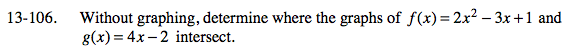### Home > A2C > Chapter 13 > Lesson 13.2.1 > Problem13-106

13-106.Substitute 4x − 2 for y in the first equation.
y = 2x2 − 3x + 1
y = 4x − 2

Set the equation equal to 0 and factor.

$x=\frac{1}{2}\text{ and }x=3$

Substitute each value of x into the second equation and solve for y.

$\text{They intersect at }\left(\frac{1}{2}, 0\right)\text{ and }(3, 10)$